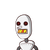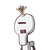# The length and breadth of a rectangle are in the ratio 3:2. If the area of the rectangle is 216sq.m, then find the length of

The length and breadth of a rectangle are in the ratio 3:2. If the area of the
rectangle is 216sq.m, then find the length of the rectangle and also find
the perimeter.

### 2 thoughts on “The length and breadth of a rectangle are in the ratio 3:2. If the area of the<br /> rectangle is 216sq.m, then find the length of”

1.Step-by-step explanation:

Let the dimensions of the Rectange be x

Area of Rectangle = l × b

216 m^2 = 3x × 2x

216 m^2 = 6x^2

216/6 = x^2

36 = x^2

6 = x

Length = 3x = 3 × 6 = 18

Breadth = 2x = 2 × 6 = 12

Perimeter of Rectangle = 2 ( l + b )

Perimeter of Rectangle = 2 ( 18 + 12 )

Perimeter of Rectangle = 2 × 30

Perimeter of Rectangle = 60 m

## Hope it helps!!

2.Let the length be 3x and breadth be 2x.

We know that,

Substituting the values :-

→ 216 = 3x × 2x

→ 216 = 6x²

→ x² = 216 / 6

→ x² = 36

→ x = 6

• Length = 6 × 3 = 18 m
• Breadth = 6 × 2 = 12 m

→ Perimeter = 2 ( Length + Breadth )

→ P = 2 ( 18 + 12 )

→ P = 2 ( 30 )

→ P = 60